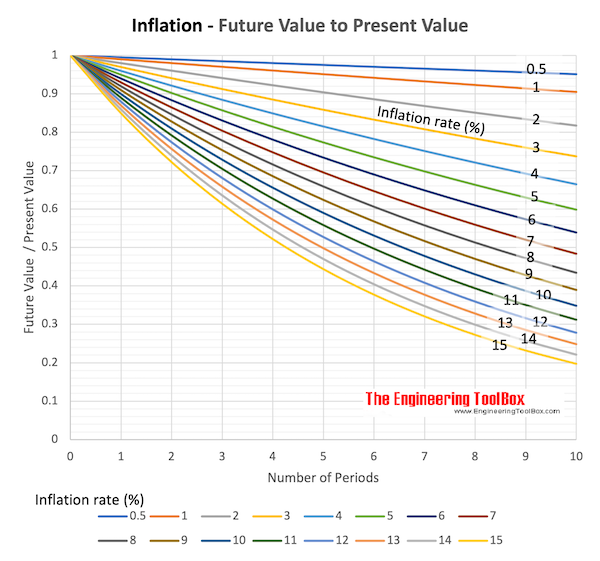Engineering ToolBox - Resources, Tools and Basic Information for Engineering and Design of Technical Applications!

# Inflation Rate

## Future value of money vs. inflation.

• An inflation with rising prices will decrease the value of money over time
• A deflation - the opposite of inflation or negative inflation - with decreasing prices will increase the value of money over time

### Inflation Rate

The future value of money after periods with uniform inflation rates can be expressed as

F = P (1 - i)n          (1)

where

F = future value

P = present value

i = average inflation (or deflation) rate per period (positive for inflation, negative for deflation)

n = number of periods

### Example - Inflation and Future Value

The future value of an amount of 100 after 10 periods and 4% of inflation rate can be calculated as

F = 100 (1 - 0.04)10 =

= 66.5

### Future Value of Present Money due to Inflation Rate - Calculator Table

This calculated table visualizes the decrease in future value of present money due to inflation rate and time.

Present Value of Money

Start Year

End Year

Max. Inflation Rate (%)

###Download and print Inflation chart - Future Value vs. Present Value and Inflation Rate

### Variable Inflation Rate

The future value of money after periods with variable inflation rates can be calculated as

F = P (1 - i1) (1 - i2) ...... (1 - in)                  (2)

where

i1..n = inflation rates of terms

The average inflation rate for all periods can be calculated as

(1 - ia)n = (1 - i1) (1 - i2) ...... (1 - in)                (3)

or

ia = 1 - [(1 - i1) (1 - i2) ...... (1 - in)]1/n                    (3b)

where

ia = average inflation rate

## Related Topics

• Economics - Engineering economics - cash flow diagrams, present value, discount rates, internal rates of return - IRR, income taxes, inflation.

## Engineering ToolBox - SketchUp Extension - Online 3D modeling!

Add standard and customized parametric components - like flange beams, lumbers, piping, stairs and more - to your Sketchup model with the Engineering ToolBox - SketchUp Extension - enabled for use with the amazing, fun and free SketchUp Make and SketchUp Pro .Add the Engineering ToolBox extension to your SketchUp from the SketchUp Pro Sketchup Extension Warehouse!

Translate

## Privacy

We don't collect information from our users. Only emails and answers are saved in our archive. Cookies are only used in the browser to improve user experience.

Some of our calculators and applications let you save application data to your local computer. These applications will - due to browser restrictions - send data between your browser and our server. We don't save this data.

## Citation

• Engineering ToolBox, (2008). Inflation Rate. [online] Available at: https://www.engineeringtoolbox.com/inflation-rate-d_1236.html [Accessed Day Mo. Year].

Modify access date.

. .

#### Scientific Online Calculator6 24